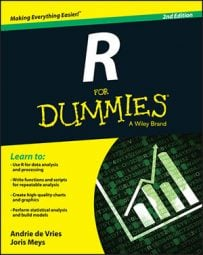##### R For DummiesWhen you are trying to create tables from a matrix in R, you end up with trial.table. The object trial.table looks exactly the same as the matrix trial, but it really isn’t. The difference becomes clear when you transform these objects to a data frame. Take a look at the outcome of this code:

```> trial.df <- as.data.frame(trial)
> str(trial.df)
‘data.frame’: 2 obs. of 2 variables:
\$ sick  : num 34 11
\$ healthy: num 9 32```

Here you get a data frame with two variables (sick and healthy) with each two observations. On the other hand, if you convert the table to a data frame, you get the following result:

```> trial.table.df <- as.data.frame(trial.table)
> str(trial.table.df)
‘data.frame’: 4 obs. of 3 variables:
\$ Var1: Factor w/ 2 levels "risk","no_risk": 1 2 1 2
\$ Var2: Factor w/ 2 levels "sick","healthy": 1 1 2 2
\$ Freq: num 34 11 9 32```

The as.data.frame() function converts a table to a data frame in a format that you need for regression analysis on count data. If you need to summarize the counts first, you use table() to create the desired table.

Now you get a data frame with three variables. The first two — Var1 and Var2 — are factor variables for which the levels are the values of the rows and the columns of the table, respectively. The third variable — Freq — contains the frequencies for every combination of the levels in the first two variables.

In fact, you also can create tables in more than two dimensions by adding more variables as arguments, or by transforming a multidimensional array to a table using as.table(). You can access the numbers the same way you do for multidimensional arrays, and the as.data.frame() function creates as many factor variables as there are dimensions.